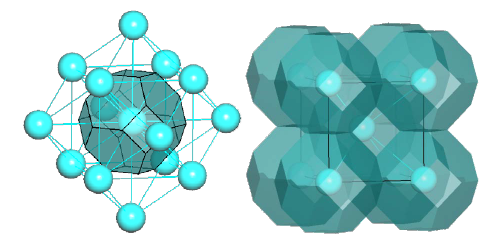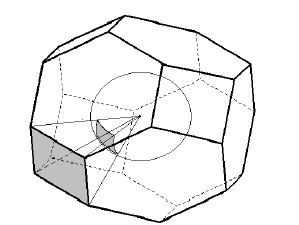﻿ ToposPro » Details of the AutoCN algorithms.
Details of the AutoCN algorithms.

The Domains algorithm mimics the way of how the crystal chemist determines interatomic interactions and classifies them, however, instead of the human intuition, it rests upon a strict sequence of steps, and clearly defined numerical parameters.

1. For each non-equivalent atom A its Voronoi-Dirichlet polyhedron (VDP, Fig. 1) is constructed, and only the atoms X that are direct neighbors of A (the lines connecting them with A cross the corresponding VDP faces) are taken into account at the subsequent steps.Figure 1: Voronoi-Dirichlet polyhedron (left) and partition (right) for the body-centered lattice.

2. For all contacts A-Xi the solid angles Ωi of pyramids with the basal VP face corresponding to the A-Xi contact (Fig. 2) are computed. If Ωi < MinOm (see the AutoCN parameters; default value is 1.5% of the total solid angle 4π steradian) the contact is referred to as non-valence and its classification to H bonds, halogen bonds, or van der Waals interactions is performed according to additional geometrical criteria.Figure 2: The solid angle (Ω) of the VDP pyramid in the body-centered lattice based on the shaded face is equal to the shaded segment of the unit sphere cut off by the pyramid with the VDP atom at the vertex and the face in the base.

3. For each atom type two kinds of radii are determined: Slater's radius, rs, (it is a constant that is predetermined in the ToposPro parameters) and averaged radius of spherical domain (Rsd, the radius of a sphere of the VDP volume, averaged over all atoms of a given sort in the structure). The former radius characterizes the size of the atom accepted in crystal chemistry; the latter one estimates the real size of a given atom depending on the atoms in the particular structure.

4. For each pair A-X the reference distance D(A-X) is determined, which is equal to the minimum of two sums: Rsd(A) + Rsd(X) or [rs(A) + rs(X)](1 + δ), where δ = 0.25 by default (it can be changed in the ToposPro parameters; Extra Dist. value). D(A-X) means the maximal distance, at which the contact A-X should be considered as strong (valence) bond.

5. The D(A-X) values are modified to take into account the features of the structure. For this purpose, if A is a metal atom or both A and X are non-metals, all distances d(A-X) are grouped if they do not differ more than the Min. Interval value (see the AutoCN parameters; default value is 0.3 Å). If after this the D(A-X) value falls within one of the groups, it increases up to the maximal value of the group. This operation allows to avoid the situation when the contacts, whose distances are very close to but larger than D(A-X), are not considered as bonds.

6. If A is a metal and with the D(A-X) values obtained at the previous steps it does not form any valence bond, the corresponding D(A-X) value is increased up to the maximal distance value of the closest group of contacts. As a result the closest contacts A-X will be considered as valence (the metal atom cannot have zero coordination number).

7. If both A and X are metals and at least one of them is s or p metal then the valence contact between them can exist only if there no valence bonds between them and non-metal atoms, otherwise D(A-X) is specified to zero. This prevents s or p metal atoms from valence bonds with other metals except the structures of intermetallic compounds.

8. If A is a metal and X is a boron atom connected to O or F atoms then the bond A-X (if any) is broken (D(A-X) = 0).

9. If there are several non-equivalent pairs (A, X) and, hence, several Di(A-X) values have been chosen at the previous steps, then the maximal Di(A-X) is chosen as the final reference distance for all contacts of a particular A-X type.

10. All contacts A-X with d(A-X) < D(A-X) and with solid angle of a pyramid with the basal VDP face corresponding to the A-X contact (Fig. 2) Ωi > MinOm (see the AutoCN parameters; default value is 1.5% of the total solid anglesteradian) are considered as valence. Other contacts are assumed non-valence and their classification to H bonds, halogen bonds, or van der Waals interactions is performed according to additional geometrical criteria.

Thus, in contrast to standard crystallochemical approach, when the bonds A-Xi are determined according to just one fixed parameter D(A-Xi), in the Domains method, three criteria are used: (i) the sign of direct neighbor, (ii) the solid angle value ΩI (both of them estimate the screening of Xi from A by other atoms), and (iii) the floating D(A-Xi), which essentially depends on a particular structure and takes into account all non-equivalent contacts A-Xi in it. The Domains algorithm was tested for thousands of structures from the CSD and ICSD, and showed a good agreement with the chemical models.

The Solid Angles algorithm is useful for intermetallic compounds; here Ωi > MinOm is the only criterion to select connected net nodes from surrounding ones; it;

The Ranges algorithm is mainly intended for artificial nets with nodes, not atoms; the nodes are considered connected if the distance between them falls into specified range(s); no VDPs are constructed in this case. If the user does not specify the distance range the standard distance criterion will be used: a contact A–B is stored in adjacency matrix if its distance is less than [rs(A) + rs(X)](1 + δ).

With these three algorithms the user can compute adjacency matrices in an automated mode, which is very important for the analysis of large amounts of crystal structures.